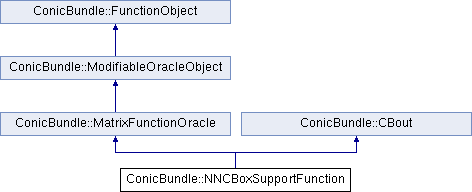ConicBundle
ConicBundle::NNCBoxSupportFunction Class Reference

general purpose implementation of MatrixFunctionOracle as explained in implemention of MatrixFunctionOracle (NNCBoxSupportFunction) (see, however, the likely better choice of a BoxOracle) More...

#include <NNCBoxSupportFunction.hxx>

Inheritance diagram for ConicBundle::NNCBoxSupportFunction:Public Member Functions

Initialization
NNCBoxSupportFunction (const CH_Matrix_Classes::Matrix &lb, const CH_Matrix_Classes::Matrix &ub, const CBout *cb=0, int incr=-1)
intialize with lower bounds vector lb and upper bounds vector ub (and output options), both must be column vectors of the same length and lb<=ub componentwise, length 0 results in objective value 0

~NNCBoxSupportFunction ()

Implementations of MatrixFunctionOracle routines
int evaluate (const CH_Matrix_Classes::Matrix &current_point, CH_Matrix_Classes::Real relprec, CH_Matrix_Classes::Real &objective_value, std::vector< Minorant *> &minorants, PrimalExtender *&primal_extender)
see MatrixFunctionOracle::evaluate()

virtual int apply_modification (const OracleModification &oracle_modification, const CH_Matrix_Classes::Matrix *new_center, const CH_Matrix_Classes::Matrix *old_center, bool &discard_objective_in_center, bool &discard_model, bool &discard_aggregates, MinorantExtender *&minorant_extender)
see MatrixFunctionOracle::apply_modification() for the general use, here oracle_modification has a special role if it can be cast to an AMFModification More...

virtual bool check_correctness () const
see MatrixFunctionOracle::check_correctness() (true only needed for debugging)

routines for querying data of the problem
const CH_Matrix_Classes::Matrixget_lower_bounds ()
returns the column vector of lower bounds

const CH_Matrix_Classes::Matrixget_upper_bounds ()
retunrs the column vector of upper bounds

routines for supporting input and output
void set_out (std::ostream *o=0, int pril=1)
see ConicBundle::CBout

void set_cbout (const CBout *cb, int incr=-1)
see ConicBundle::CBout

std::ostream & print_problem_data (std::ostream &out) const
write the problem description to out so that it can be read again by read_problem_data()

clear() and read the problem from in in the format written by print_problem_data()

std::ostream & print_problem_data_to_mfile (std::ostream &out, CH_Matrix_Classes::Integer blocknr) const
undocumented highly volatile variant for external testingPublic Member Functions inherited from ConicBundle::ModifiableOracleObject
virtual ~ModifiableOracleObject ()
virtual destructorPublic Member Functions inherited from ConicBundle::CBout
void clear_cbout ()
reset to default settings (out=0,print_level=1)

CBout (const CBout *cb=0, int incr=-1)
calls set_cbout

CBout (std::ostream *outp, int pl=1)
initialize correspondingly

CBout (const CBout &cb, int incr=0)
copy constructor

virtual bool cb_out (int pl=-1) const
Returns true if out!=0 and (pl<print_level), pl<0 should be used for WARNINGS and ERRORS only, pl==0 for usual output.

std::ostream & get_out () const
If cb_out() returned true, this returns the output stream, but it will abort if called with out==0.

std::ostream * get_out_ptr () const
returns the pointer to the output stream

int get_print_level () const
returns the print_level

virtual int mfile_data (std::ostream &out) const
writes problem data to the given outstream

Private Member Functions

int apply_modification (const NNCBoxSupportModification &mod)
applies the NNCBoxSupportModfication mod to the current function

Private Attributes

CH_Matrix_Classes::Matrix lb
column vector of lower bounds

CH_Matrix_Classes::Matrix ub
column vector of upper bounds

Detailed Description

general purpose implementation of MatrixFunctionOracle as explained in implemention of MatrixFunctionOracle (NNCBoxSupportFunction) (see, however, the likely better choice of a BoxOracle)

The documentation for this class was generated from the following file: